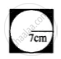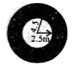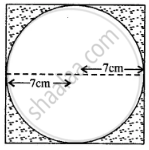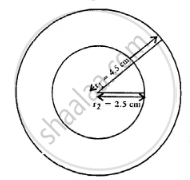# Find the Area of the Shaded Portion in Each of the Following Diagrams : - Mathematics

Sum

Find the area of the shaded portion in each of the following diagrams :

(i)(ii)#### Solution

(i)Radius of circle, r = 7 cm

∴ Side of square = 7 + 7 = 14 cm

Area of circle = pir^2

= 22/7 xx 7xx 7

= 154 cm2

Area of square = 14 xx 14

= 196 cm2

∴ Area of shaded portion = 196 - 154

= 42 cm2

(ii)

r_1 = 4.5m

r_2 = 2.5m∴ Area of shaded portion = pir_1^2 - pir_2^2

= pi[r_1^2 - r_2^2] = 22/7[(4.5)^2 - (2.5)^2]

= 22/7 xx (4.5 + 2.5) (4.5 - 2.5)

= 22/7 xx 14 = 44 cm2

Concept: Area of Circle
Is there an error in this question or solution?
Chapter 20: Area of a Trapezium and a Polygon - Exercise 20 (D) [Page 234]

#### APPEARS IN

Selina Concise Mathematics Class 8 ICSE
Chapter 20 Area of a Trapezium and a Polygon
Exercise 20 (D) | Q 6 | Page 234
Share